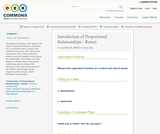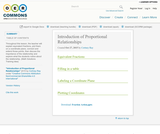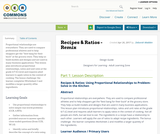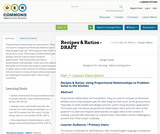# Search Results (10)

View
Selected filters:
• Proportional Relationships
RemixConditions of Use:
Remix and Share
Rating

Throughout this lesson, the teacher will explain equivalent fractions, plot them on a coordinate plane, connect and extend those points, then discuss the importance of the relationship and explore what the students notice about the relationship. This lesson can help depend understanding of equivalent fractions as well as deepen the discussion into slope being similar to the fraction that we gave at the beginning of the lesson. (Math Solutions Training idea)

Subject:
Mathematics
Material Type:
Activity/Lab
Author:
Cortney Ray
08/08/2019Conditions of Use:
Remix and Share
Rating

Throughout this lesson, the teacher will explain equivalent fractions, plot them on a coordinate plane, connect and extend those points, then discuss the importance of the relationship and explore what the students notice about the relationship. (Math Solutions Training idea)

Subject:
Mathematics
Material Type:
Activity/Lab
Author:
Cortney Ray
01/28/2016Conditions of Use:
Remix and Share
Rating

Four full-year digital course, built from the ground up and fully-aligned to the Common Core State Standards, for 7th grade Mathematics. Created using research-based approaches to teaching and learning, the Open Access Common Core Course for Mathematics is designed with student-centered learning in mind, including activities for students to develop valuable 21st century skills and academic mindset.

Subject:
Mathematics
Material Type:
Full Course
Provider:
Pearson
10/06/2016Conditions of Use:
Remix and Share
Rating

Proportional Relationships

Type of Unit: Concept

Prior Knowledge

Students should be able to:

Understand what a rate and ratio are.
Make a ratio table.
Make a graph using values from a ratio table.

Lesson Flow

Students start the unit by predicting what will happen in certain situations. They intuitively discover they can predict the situations that are proportional and might have a hard time predicting the ones that are not. In Lessons 2–4, students use the same three situations to explore proportional relationships. Two of the relationships are proportional and one is not. They look at these situations in tables, equations, and graphs. After Lesson 4, students realize a proportional relationship is represented on a graph as a straight line that passes through the origin. In Lesson 5, they look at straight lines that do not represent a proportional relationship. Lesson 6 focuses on the idea of how a proportion that they solved in sixth grade relates to a proportional relationship. They follow that by looking at rates expressed as fractions, finding the unit rate (the constant of proportionality), and then using the constant of proportionality to solve a problem. In Lesson 8, students fine-tune their definition of proportional relationship by looking at situations and determining if they represent proportional relationships and justifying their reasoning. They then apply what they have learned to a situation about flags and stars and extend that thinking to comparing two prices—examining the equations and the graphs. The Putting It Together lesson has them solve two problems and then critique other student work.

Gallery 1 provides students with additional proportional relationship problems.

The second part of the unit works with percents. First, percents are tied to proportional relationships, and then students examine percent situations as formulas, graphs, and tables. They then move to a new context—salary increase—and see the similarities with sales taxes. Next, students explore percent decrease, and then they analyze inaccurate statements involving percents, explaining why the statements are incorrect. Students end this sequence of lessons with a formative assessment that focuses on percent increase and percent decrease and ties it to decimals.

Students have ample opportunities to check, deepen, and apply their understanding of proportional relationships, including percents, with the selection of problems in Gallery 2.

Subject:
Mathematics
Ratios and Proportions
Material Type:
Unit of Study
Provider:
PearsonConditions of Use:
Remix and Share
Rating

Students explore the idea that not all straight lines are proportional by comparing a graph representing a stack of books with a graph representing a stack of cups. They recognize that all proportional relationships are represented as a straight line that passes through the origin.Key ConceptsNot all graphs of straight lines represent proportional relationships.There are three ways to tell whether a relationship between two varying quantities is proportional:The graph of the relationship between the quantities is a straight line that passes through the point (0, 0).You can express one quantity in terms of the other using a formula of the form y = kx.The ratios between the varying quantities are constant.Goals and Learning ObjectivesUnderstand when a graph of a straight line is and when it is not a proportional relationship.Recognize that a proportional relationship is shown on a graph as a straight line that passes through the origin (0, 0).Make a table of values to represent two quantities that vary.Graph a table of values representing two quantities that vary.Describe what each variable and number in a formula represents.

Subject:
Ratios and Proportions
Material Type:
Lesson Plan
Provider:
PearsonConditions of Use:
Remix and Share
Rating

Students interpret verbal descriptions of situations and determine whether the situations represent proportional relationships.Key ConceptsIn a proportional relationship, there has to be some value that is constant.There are some relationships in some situations that can never be proportional.Goals and Learning ObjectivesIdentify verbal descriptions of situations as being proportional relationships or notUnderstand that some relationships can never be proportionalUnderstand that for two variable quantities to be proportional to one another, something in the situation has to be constant

Subject:
Ratios and Proportions
Material Type:
Lesson Plan
Provider:
PearsonConditions of Use:
Remix and Share
Rating

Students watch a video showing three different ways to solve a problem involving a proportional relationship, and then they use each method to solve a similar problem. Students describe each approach, including the mathematical terms associated with each.Key ConceptsThree methods for solving problems involving proportional relationships include:Setting up a proportion and solving for the missing valueFinding the unit rate and multiplyingWriting and solving a formula using the constant of proportionalityGoals and Learning ObjectivesSolve a problem involving a proportional relationship in three different ways: set up a proportion and solve for a missing value, use a unit rate, and use the constant of proportionality to write and solve a formula.

Subject:
Ratios and Proportions
Material Type:
Lesson Plan
Provider:
Pearson
RemixConditions of Use:
Remix and Share
Rating

Proportional relationships are everywhere. They are used to compare professional athletes and to help shoppers get the “best bang for their buck” at the grocery store. They help us build models and designs and are used in many business applications. This lesson plan introduces proportional relationships, ratios and unit rates at the grade 6/7 (C) level and requires adult learners to apply ratios in the context of cooking. The bonus challenge: the learner completes Worksheet 2 and modifies a larger quantity ofthe ingredients.

Subject:
English Language Arts
Mathematics
Material Type:
Homework/Assignment
Lesson
Author:
08/08/2019
RemixConditions of Use:
No Strings Attached
Rating

Proportional relationships are everywhere. They are used to compare professional athletes and to help shoppers get the “best bang for their buck” at the grocery store. They help us build models and designs and are used in many business applications. This lesson plan introduces proportional relationships, ratios and unit rates at the grade 6/7 (C) level and requires adult learners to identify and compare ratios using the Padlet application.

Subject:
Mathematics
Material Type:
Assessment
Lesson
Author:
Amanda Weber
08/08/2019Conditions of Use:
No Strings Attached
Rating

Proportional relationships are everywhere. They are used to compare professional athletes and to help shoppers get the “best bang for their buck” at the grocery store. They help us build models and designs and are used in many business applications. This lesson plan introduces proportional relationships, ratios and unit rates at the grade 6/7 (C) level and requires adult learners to apply ratios in the context of cooking. Learners will need learn to research basic information and be challenged to present their “final recipe” creatively.

Subject:
English Language Arts
Mathematics
Material Type:
Lesson
Author:
Carolyn Wilson